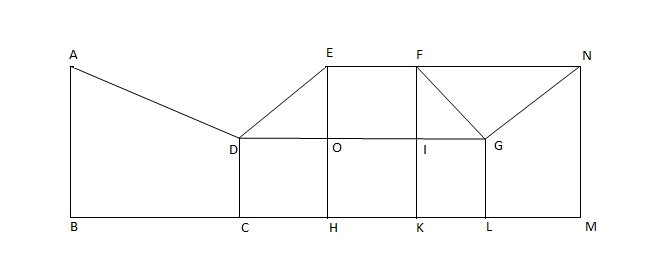# CAT 2002 Question Paper

Instructions

A boy is asked to put one mango in a basket when ordered 'One', one orange when ordered 'Two', one apple when ordered 'Three', and is asked to take out from the basket one mango and an orange when ordered 'Four'.

A sequence of orders is given as: 1 2 3 3 2 1 4 2 3 1 4 2 2 3 3 1 4 1 1 3 2 3 4

Question 1

Question 2

# How many total fruits will be in the basket at the end of the above order sequence?

Instructions

Directions for the next two questions: Answer the questions based on the following information.

Each of the 11 letters A, H, I, M, O, T, U, V, W, X and Z appears same when looked at in a mirror. They are called symmetric letters. Other letters in the alphabet are asymmetric letters.

Question 3

Question 4

# How many three-letter computer passwords can be formed (no repetition allowed) with at least one symmetric letter?

Instructions

Directions for the next two questions: Answer the questions based on the following diagram

In the following diagram, $$\angle{ABC}$$ = 90° = $$\angle{DCH}$$ = $$\angle{DOE}$$ = $$\angle{EHK}$$ = $$\angle{FKL}$$ = $$\angle{GLM}$$ = $$\angle{LMN}$$

AB = BC = 2CH = 2CD = EH = FK = 2HK = 4KL = 2LM = MNQuestion 5

Question 6

# What is the ratio of the areas of the two quadrilaterals ABCD to DEFG?

Instructions

For the following questions answer them individually

Question 7

Question 8

Question 9

Question 10

OR